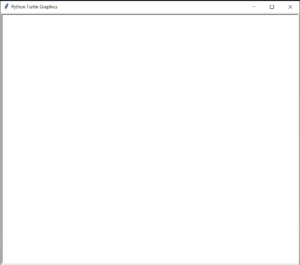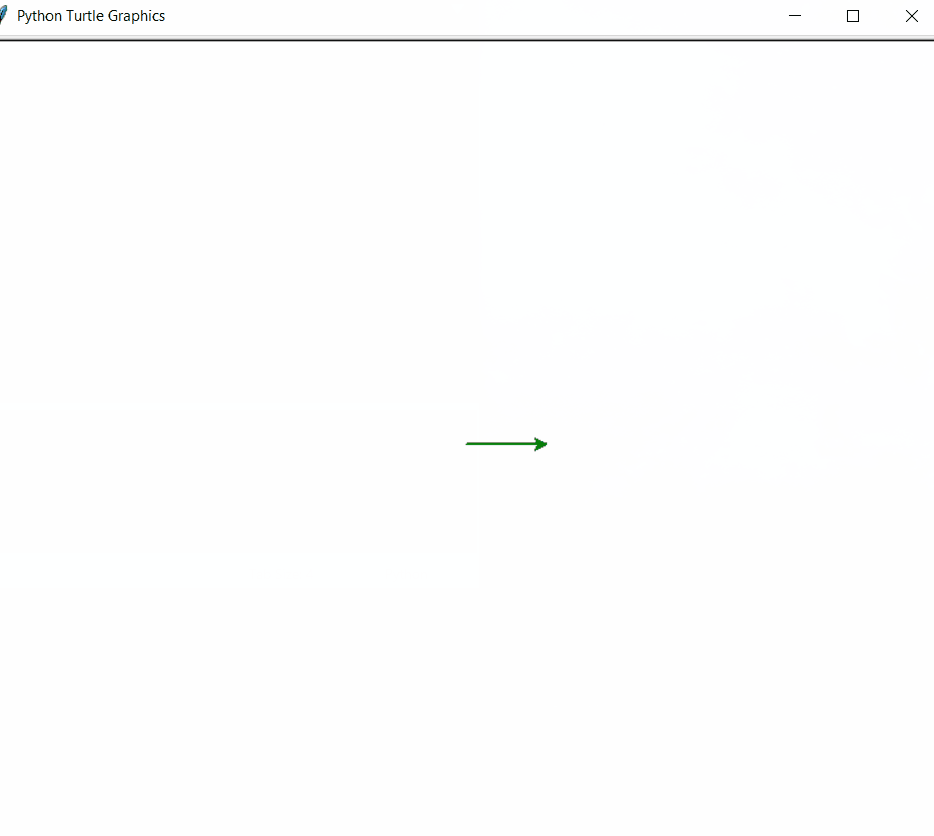Open in App
Not now

# Draw a Tic Tac Toe Board using Python-Turtle

• Difficulty Level : Medium
• Last Updated : 16 Oct, 2020

The Task Here is to Make a Tic Tac Toe board layout using Turtle Graphics in Python. For that lets first know what is Turtle Graphics.

## Turtle graphics

In computer graphics, turtle graphics are vector graphics using a relative cursor upon a Cartesian plane. Turtle is drawing board like feature which let us to command a turtle and draw using it.

Features of turtle graphics:

• backward(length): moves the pen in the backward direction by x unit.
• right(angle): rotate the pen in the clockwise direction by an angle x.
• left(angle): rotate the pen in the anticlockwise direction by an angle x.
• penup(): stop drawing of the turtle pen.
• pendown(): start drawing of the turtle pen.

### Approach

• import the turtle modules.
```import turtle

```
• Get a screen to draw on.
```ws = turtle.Screen()

```

The screen will look like this.Screen

• Define an instance for turtle.
• Here, i had manually set the speed of the turtle to 2.
• For a drawing a Tic Tac Toe board first we have to make an outer Square.
• Then we will Make inner lines of the square which will ultimately make up the board.
• For inner lines i had used penup(),goto() and pendown() method to take the pen up and then take it down at certain coordinates.
• The inner lines will make up the Tic Tac Toe board perfectly.

Below is the implementation of the above approach:

## Python3

 `import` `turtle `     `# getting a Screen to work on ` `ws``=``turtle.Screen()`   `# Defining Turtle instance` `t``=``turtle.Turtle()`   `# setting up turtle color to green ` `t.color(``"Green"``)`   `# Setting Up width to 2` `t.width(``"2"``)`   `# Setting up speed to 2` `t.speed(``2``)`   `# Loop for making outside square of` `# length 300` `for` `i ``in` `range``(``4``):` `    ``t.forward(``300``)` `    ``t.left(``90``)`     `# code for inner lines of the square` `t.penup()` `t.goto(``0``,``100``)` `t.pendown()`   `t.forward(``300``)`   `t.penup()` `t.goto(``0``,``200``)` `t.pendown()`   `t.forward(``300``)`   `t.penup()` `t.goto(``100``,``0``)` `t.pendown()`   `t.left(``90``)` `t.forward(``300``)`   `t.penup()` `t.goto(``200``,``0``)` `t.pendown()`     `t.forward(``300``)`

Output:Turtle making Tic Tac Toe Board

My Personal Notes arrow_drop_up
Related Articles